# HTFSV: High-temperature, full-shear viscosity

#### Gokhan

The base-oil viscosity and viscosity-index improver (VII) table has just got major enhancements! Now, I have added three columns to the table for the base-oil kinematic viscosity at 40 C (BO KV40), base-oil kinematic viscosity at 100 C (BO KV100), and base-oil viscosity index (BO VI). These are typical viscosity indexes for the common base stocks: BO VI (base-oil viscosity index) = 80 - 119 (Group I/II), 120 - 129 (Group III), 126 - 139 (PAO), 130 - 150 (GTL/Group III+++), 74 - 105 (AN), 130 - 136 (POE) You can now do at least three things: (1) You can get an idea on the base-oil composition of a given oil by calculating the base-oil viscosity index (BO VI) using the density, KV40, KV100, and HTHSV values from the PDS. (2) By assuming on the base-oil composition and a value for the base-oil viscosity index (BO VI), you can calculate the HTHSV. (3) Calculate the VII content and dynamic base-oil viscosity at 150 C (BO DV150 = HTFSV). The calculated base-oil viscosity index (BO VI) for most oils are within the range one would expect from the marketed, MSDS-specified, or speculated compositions of the oils. However, Mobil 1 High-Mileage (M1 HM) oils are a peculiar case with the base-oil viscosity index (BO VI) coming out of the calculator much lower than one would naÃ¯vely expect. A possible explanation of this M1 HM anomaly is that M1 HM base oils contain a very high amount of alkylated naphthalene (AN) base stocks. 4.7 cST AN base stock (Synesstic 5) has VI = 74 and 12.4 cSt AN base stock (Synesstic 12) has VI = 105. These would lower the base-oil viscosity index (BO VI) substantially. We already knew that all Mobil 1 oils except the Euro (FS and ESP) flavors contained AN base stocks and the Euro (FS and ESP) flavors contained POE base stocks. The results of the calculator are suggesting that the amount of the AN base stocks in the M1 HM oils are much higher than in other M1 oils. In fact, AN base stocks are used as seal swellers and engine-deposit cleaners, which are therefore very suitable for high-mileage oils: Information by TRiiSO on Exxon Mobil Synesstic AN base stocks It looks like the base-oil viscosity and VII calculator has discovered something that was previously unknown to everyone -- namely the generous use of the AN base stocks in the M1 HM oils! Enjoy the latest table. You can download the Google Sheet if you like. Make sure to read the notes at the bottom. Last but not least, two caveats: (1) The calculator assumes olefin copolymer (OCP) VII, which is by far most popular in modern oils because of its resistance to form engine and turbocharger deposits and sludge. It wouldn't work with other types of VII such as star/Asteric. (2) The calculation is only as good as the input data provided. It is especially sensitive to HTHSV input data and some manufacturers are not good at reporting accurate values for it. With that said, here is the link to the Google Sheet and an image of the table. It shouldn't be used as a rating of the oils but rather to understand their base-oil and VII composition for a more informed oil selection: Estimated base-oil dynamic viscosit... improver (VII) content of selected oils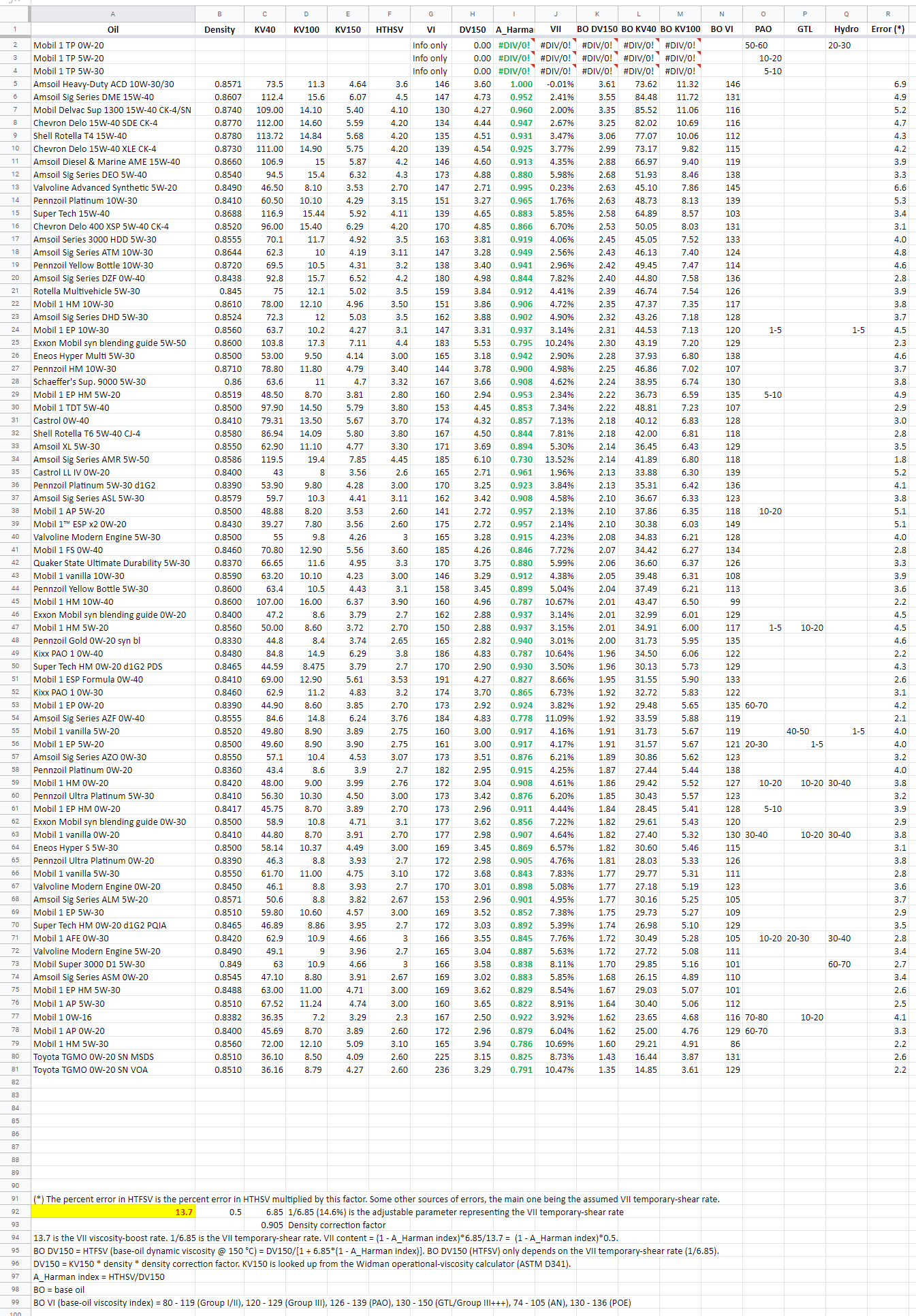#### Gokhan

The base-oil viscosity and viscosity-index improver (VII) table has just got major enhancements! Now, I have added three columns to the table for the base-oil kinematic viscosity at 40 C (BO KV40), base-oil kinematic viscosity at 100 C (BO KV100), and base-oil viscosity index (BO VI). These are typical viscosity indexes for the common base stocks: BO VI (base-oil viscosity index) = 80 - 119 (Group I/II), 120 - 129 (Group III), 126 - 139 (PAO), 130 - 150 (GTL/Group III+++), 74 - 105 (AN), 130 - 136 (POE) You can now do at least three things: (1) You can get an idea on the base-oil composition of a given oil by calculating the base-oil viscosity index (BO VI) using the density, KV40, KV100, and HTHSV values from the PDS. (2) By assuming on the base-oil composition and a value for the base-oil viscosity index (BO VI), you can calculate the HTHSV. (3) Calculate the VII content and dynamic base-oil viscosity at 150 C (BO DV150 = HTFSV). The calculated base-oil viscosity index (BO VI) for most oils are within the range one would expect from the marketed, MSDS-specified, or speculated compositions of the oils. However, Mobil 1 High-Mileage (M1 HM) oils are a peculiar case with the base-oil viscosity index (BO VI) coming out of the calculator much lower than one would naÃ¯vely expect. A possible explanation of this M1 HM anomaly is that M1 HM base oils contain a very high amount of alkylated naphthalene (AN) base stocks. 4.7 cST AN base stock (Synesstic 5) has VI = 74 and 12.4 cSt AN base stock (Synesstic 12) has VI = 105. These would lower the base-oil viscosity index (BO VI) substantially. We already knew that all Mobil 1 oils except the Euro (FS and ESP) flavors contained AN base stocks and the Euro (FS and ESP) flavors contained POE base stocks. The results of the calculator are suggesting that the amount of the AN base stocks in the M1 HM oils are much higher than in other M1 oils. In fact, AN base stocks are used as seal swellers and engine-deposit cleaners, which are therefore very suitable for high-mileage oils: Information by TRiiSO on Exxon Mobil Synesstic AN base stocks It looks like the base-oil viscosity and VII calculator has discovered something that was previously unknown to everyone -- namely the generous use of the AN base stocks in the M1 HM oils! Enjoy the latest table. You can download the Google Sheet if you like. Make sure to read the notes at the bottom. Last but not least, two caveats: (1) The calculator assumes olefin copolymer (OCP) VII, which is by far most popular in modern oils because of its resistance to form engine and turbocharger deposits and sludge. It wouldn't work with other types of VII such as star/Asteric. (2) The calculation is only as good as the input data provided. It is especially sensitive to HTHSV input data and some manufacturers are not good at reporting accurate values for it. With that said, here is the link to the Google Sheet and an image of the table. It shouldn't be used as a rating of the oils but rather to understand their base-oil and VII composition for a more informed oil selection: Estimated base-oil dynamic viscosit... improver (VII) content of selected oils#### Gokhan

I set the calibration parameter to 10.5/2 (older value was 13.7/2) after comparing it against actual test oils. Here is the theory behind: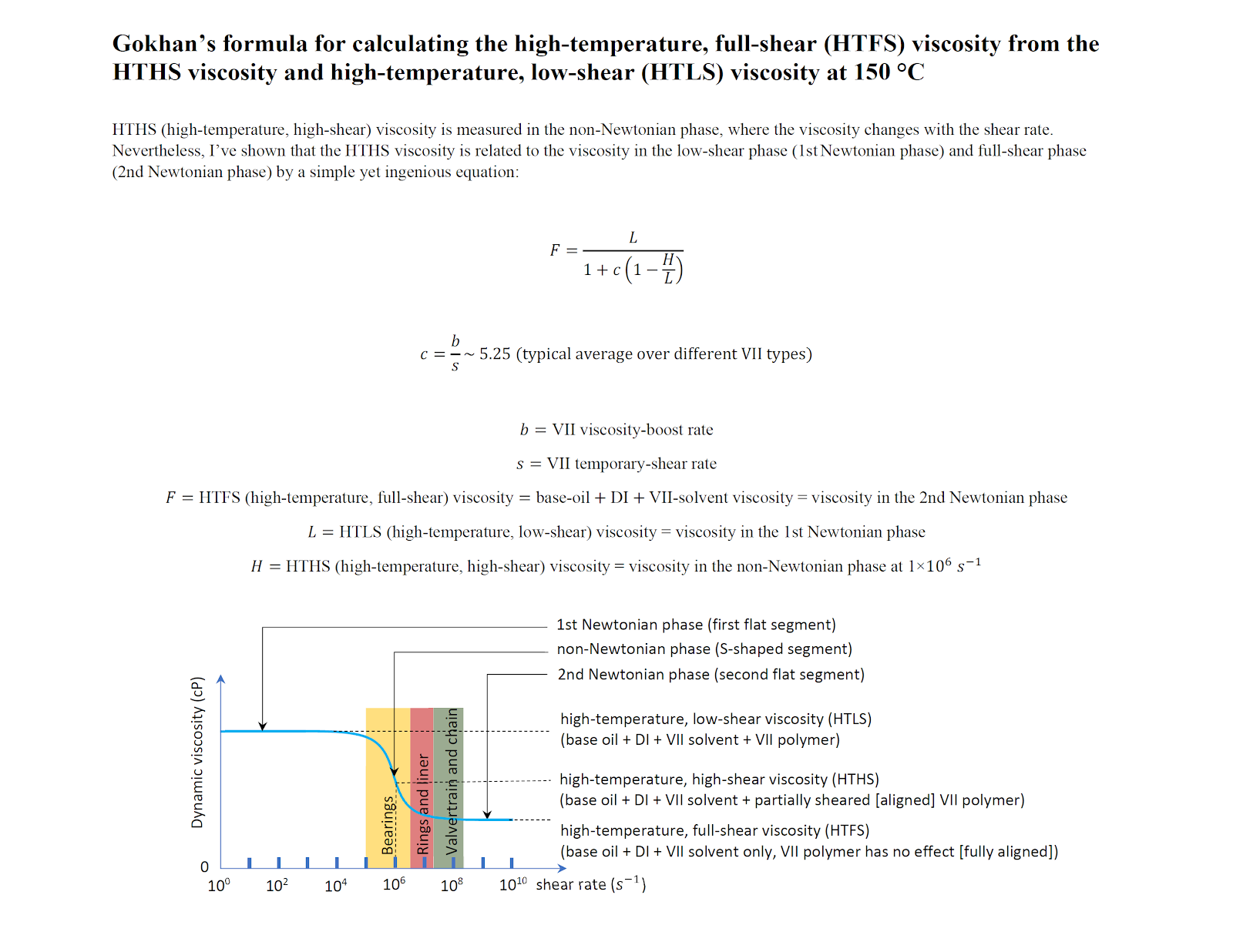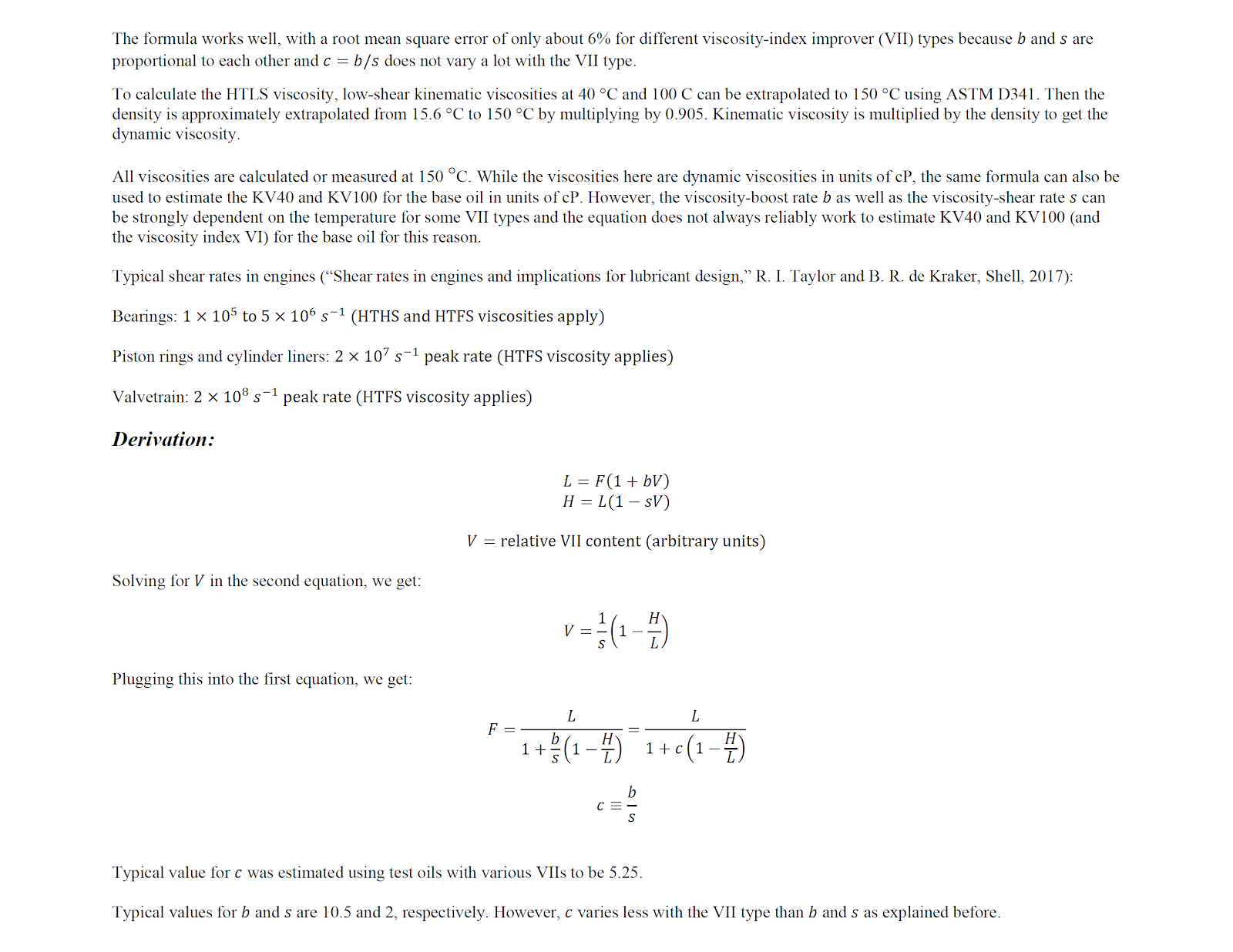The average error in HTFS for different VII types is only 6%. However, I didn't study the error in ASTM D341 and density extrapolation, as the paper had the dynamic-viscosity values directly but not the density values.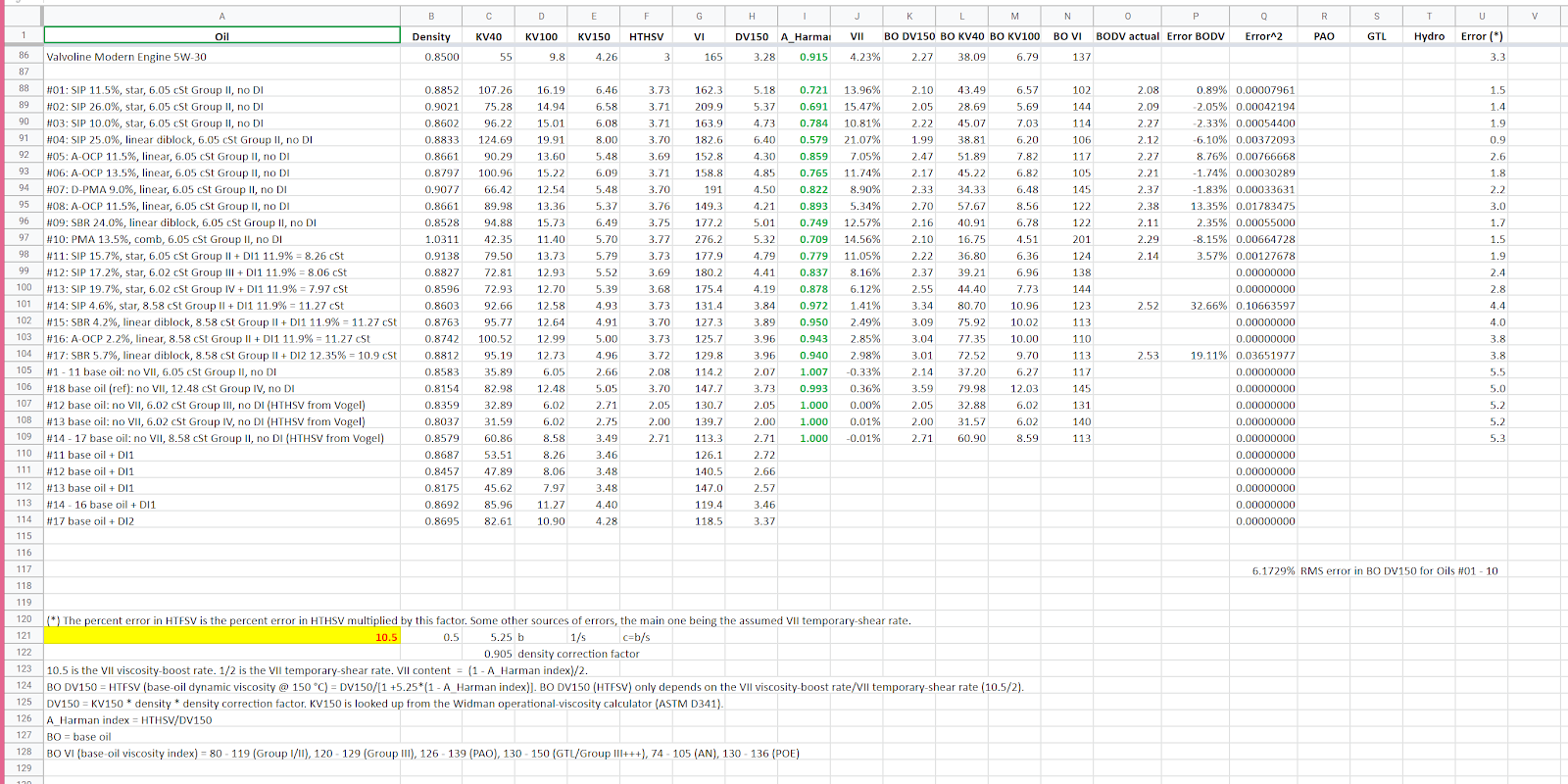#### Gokhan

Here is the latest table with the calibration constant set to 10.5 after calibrating it against the test oils in the shear-thinning paper by Hugh Spikes et al. HTFS = BO DV150 = base oil + fully sheared DI + VII solvent viscosity @ 150 Â°C (full temporary shear of the oil, VII polymer has no effect on viscosity) Note that I didn't fill in the base-oil viscosity index (BO VI) values because they could be inaccurate. However, you can easily calculate them using the BO KV40 and BO KV100 in the Widman viscosity-index calculator. You can download the Excel sheet here: https://docs.google.com/spreadsheets/d/1oIYJP_5lgdt9l-_5n_ftKL5ScaaeY0MErFRothajZos/edit?usp=sharing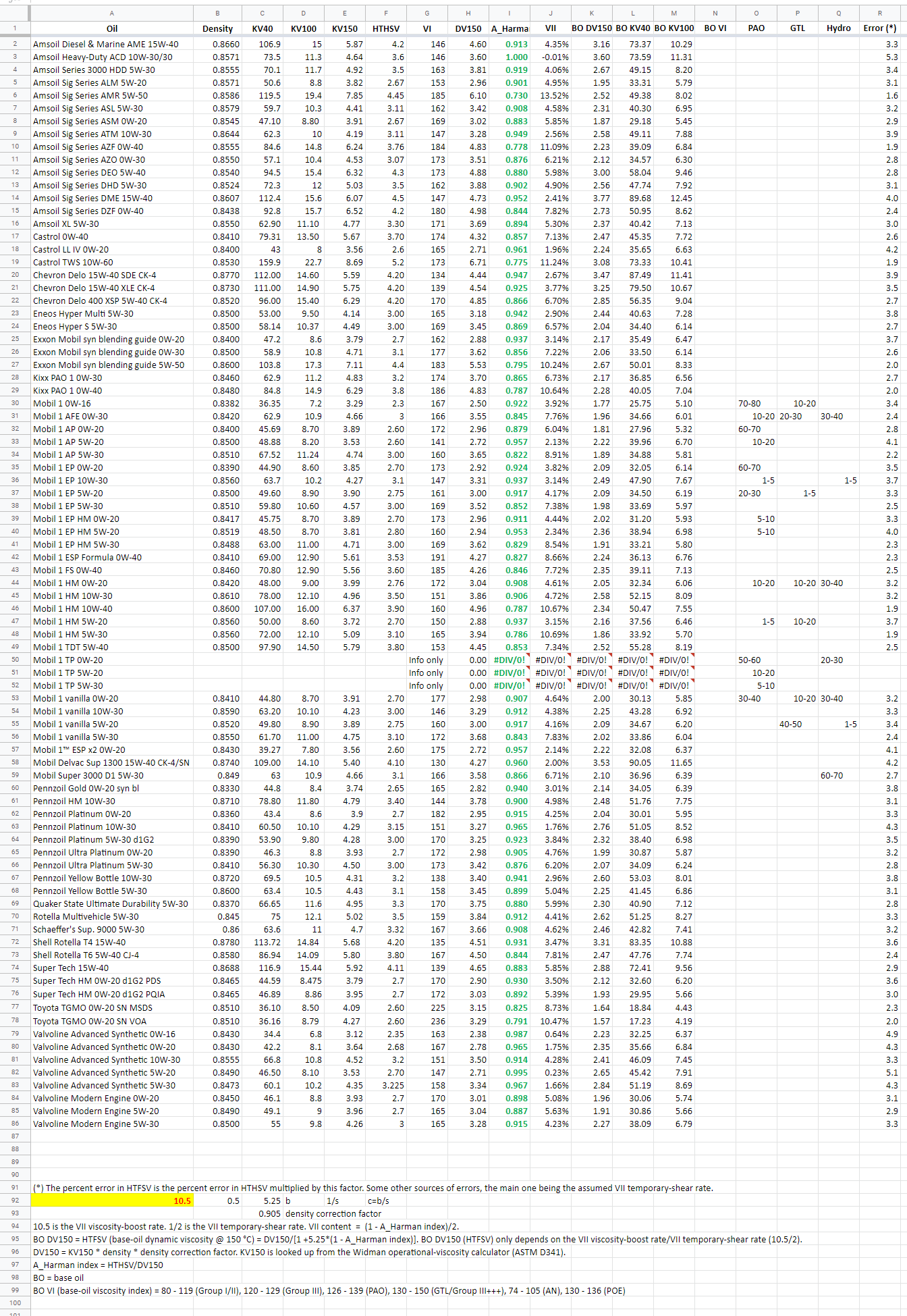#### Gokhan

I will explain how to calculate the HTFS (base-oil viscosity at 150 Â°C) using an example. You need to know: density15.6 (density @ 15.6 Â°C) KV40 (kinematic viscosity at 40 Â°C) KV100 (kinematic viscosity at 100 Â°C) HTHS viscosity @ 150 Â°C You intend to calculate: HTFS viscosity @ 150 Â°C = (viscosity of the base oil and additives but without the VII boost @ 150 Â°C = viscosity under full shear at 150 Â°C) Example: Mobil 1 Extended Performance 0W-20 SN PLUS density15.6 = 0.839 g/mL KV40 = 44.90 cSt KV100 = 8.60 cSt HTHS = 2.70 cP (values taken from the product data sheet) Step 1: Go to the Widman operational kinematic-viscosity calculator that employs ASTM D341 and calculate KV150 from KV40 and KV100: https://www.widman.biz/English/Calculators/Operational.html KV150 = 3.85 cSt (calculated by the Widman ASTM D341 calculator) Step 2: Input the density15.6, KV150 and HTHS150 into the Excel sheet (or Google sheet) linked above. The sheet will calculate HTFS viscosity = BO DV150 (base-oil dynamic viscosity at 150 Â°C) HTFS = 2.09 cP (calculated by the Excel sheet) That's it! Don't forget that the HTFS value is an estimate. Note that the Excel sheet extrapolates the density from 15.6 Â°C to 150 Â° by multiplying by 0.905. The Excel sheet also calculates the base-oil (BO) KV40 and BO KV100, from which you can calculate the BO viscosity index (VI) using a VI calculator. However, these numbers are often not accurate and use them cautiously. Here is the Widman VI calculator: https://www.widman.biz/English/Calculators/calc-visc-index.html Now that you have HTFS = BO DV150, what do you do with it? Higher HTFS will help reduce the valvetrain, timing-chain, piston-ring, and cylinder-liner wear, as these parts experience ultrahigh shear rates, at which HTFS is the applicable viscosity. Higher HTFS along with higher HTHS may also help reduce the bearing wear, as bearings can experience somewhat higher shear rates than at which HTHS is measured (1,000,000 1/second) as well. On the downside for a given HTHS viscosity, higher HTFS viscosity will make the fuel economy worse, as expected from a thicker oil over a wider shear-rate range.

#### Shannow

Gokhan, I've received a PM from a member asking how to calculate the HTHS of a mix...I use the Widman calculator, even though it's dynamic rather than kinematic...it's probably close enough for Government work. We've seen a couple of mixes that didn't quite marry up KV100 wise with the calculated figures. But in that PM conversation, I thought of your calculator, and how valuable it would be to predicting the viscometrics of a mix (I refuse to use the word blend). In theory, your calculator should be able to identify the base oil viscosities for the two oils, and the impact of the VMs on them...then mix the base oils, using well known relationships...then re-introduce the viscosity modifiers, and get back toe the actual KV100s and HTHS. Would be great if you could demonstrate it firstly with the blend guide...and I'll try to get the guy's proposed mix for you to work on.

#### Gokhan

Hi Shannow, My calculator needs these: density, KV150, and HTHS. In order to calculate a mix, say CATERHAM blend, I would do this: (1) Use my calculator to find the VII contents for the two oils (2) Calculate the weighted average of the VII contents (3) Calculate the weighted average of the density (4) Use the Widman mixing calculator to calculate the KV40 and KV100 of the mix https://www.widman.biz/English/Calculators/Mixtures.html (5) Use the Widman operational kinematic viscosity calculator to calculate the KV150 of the mix from the KV40 and KV100 of the mix https://www.widman.biz/English/Calculators/Operational.html (6) Use my calculator to adjust the HTHS manually until the VII content of the mix agrees with the weighted average VII content Here is an example: 50% Mobil 1 FS 0W-40 (HTHS = 3.6 cP) and 50% Mobil 1 EP 0W-20 (HTHS = 2.7 cP) density_mix = 0.8425 g/mL VII_mix = 5.77% KV40_mix = 56.15 cSt KV100_mix = 10.49 cSt KV150_mix = 4.61 cSt HTHSV_mix (calculator output) = 3.11 cP Note that this is an estimate only, as we don't know the exact VII types and exact values of the viscosity boost/shear (10.5/2 = 5.25 used in the calculator). So, it's not too far from the simple weighted average of the HTHS values, which is 3.15 cP. The example is in the bottom row: https://docs.google.com/spreadsheets/d/1gnOrQxsbymULx1s6_uBQi8zNHfJXg7lwwQpwzLSIWQI/edit?usp=sharing

#### Gokhan

It just occurred to me that calculating the VII content involves using the shear-rate constant s (~ 2.0) separately, which is prone to more error than using the boost/shear (b/s ~ 5.25) together, which is used for HTFS = BO DV150. That's because the variation of b/s is smaller than the individual variations of b and s with the VII type. So, instead of looking at the VII content, I would rather look at the HTFS = BO DV150, which is more accurate. In fact, that's the only thing my calculator really attempts to estimate. The VII content is more of an intermediate result in arbitrary units. For the example I gave, using the Widman mixing calculator, HTFS_mix = BO DV150_mix = 2.22 cP This gives HTHS_mix = 3.124 cP. It brings it even closer to the simple weighted average, which is 3.15 cP. Let me know what you get for your mix.

#### Gokhan

OK, I have updated my approach to calculate the HTHS of the mix/blend so that the VII content is not used for the reasons stated in the above post. Here are the new steps: My calculator needs these three things to work: density, KV150, and HTHS. Note that HTFS = BO DV150 refers to the base-oil dynamic viscosity (including the sheared additives) at 150 Â°C. Also note that DV150 = HTLS is the low-shear viscosity at 150 Â°C. In order to calculate HTHS of a mix/blend, say CATERHAM blend, I would do this: (1) Use my calculator to find the (a) DV150 (HTLS) and (b) HTFS (BO DV150) for the two oils. In other words find the low-shear and full-shear viscosities of the two oils. (2) Use my viscosity-calculator (link) to find the (a) DV150 (HTLS) and (b) HTFS (BO DV150) for the mix. (3) Calculate the density of the mix, which is the weighted average of the two densities. (4) Divide the DV150 of the mix by the density of the mix. Then divide the resultant number by 0.905. This is the KV150 of the mix. (4) Enter the density of the mix, KV150 of the mix, and a guess for the HTHS of the mix in my calculator. Check that DV150 agrees with the value you calculated in Step 2; otherwise, fix the error. (5) Adjust the HTHS manually until the HTFS (BO DV150) of the mix agrees with the value you calculated in Step 2. This is my calculator's HTHS estimate for the mix. Here is an example: 50% Mobil 1 FS 0W-40 (HTHS = 3.6 cP) and 50% Mobil 1 EP 0W-20 (HTHS = 2.7 cP) density_mix = 0.8425 g/mL DV150_mix = 3.5269 cP HTFS (BO DV150)_mix = 2.2162 cP KV150_mix = 4.6257 cSt HTHSV_mix (my calculator's output) = 3.104 cP Note that this is an estimate only, as we don't know the exact VII types and exact values of the viscosity boost/shear (10.5/2 = 5.25 used in the calculator). So, it's not too far from the simple weighted average of the HTHS values, which is 3.15 cP. The example is in the bottom row: https://docs.google.com/spreadsheets/d/1gnOrQxsbymULx1s6_uBQi8zNHfJXg7lwwQpwzLSIWQI/edit?usp=sharing

#### Boxnuts

Hi All All credit to Gokhan for his work which is way above my pay grade. However I also like doodling with spreadsheets and have an interest in viscosity temperature calcs and VI etc. I would like raise a small point. Gokhan uses a factor of 0.905 to compute density 150. But I think this is a bit high. I have looked at two sources. Firstly Exxon Mobil data for their SpectraSyn andSpectraSyn Plus PAO oils. The spec sheets published give for example for SpectraSyn Plus 4 a figure of 6.44E-4. This seems to have been used by previous posters as the temp coeff of expansion. This I think is wrong. The 6.44E-4 is given with units of g/cmÂ³/Â°C whereas a temp coeff would only have units of per Â°C. The density correction factor as Exxon call it should be used as follows. Density 15 = 0.820 g/ cmÂ³ Density t = density15 + Dcf*(15.6-t) Density 150 = 0.820 - 0.0866 = 0.733 The simple factor is therefore 0.733/0.820 = 0.894 The other oils range from 0.893 to 0.898 the higher the density the higher the factor. Secondly ASTM table 54D gives what they call the "volume correction factor" for lubricating oil. There are spreadsheets and apps on line that use this data. This data is likely to reflect basic mineral oil but despite this the VCF figures for the above PAO oils are virtually identical. If you just want an average, 0.895 would seem to be a good figure. But if you want precision I have a simple formula for your spreadsheet: VCF = 0.126*density15 + 0.791 and therefore Density 150 = density15 * VCF Sorry to go on so much about a minor point but if you are going to do spreadsheets you might as well get it right.

#### Gokhan

Hey Boxnuts, Thank you for your input. The formula you're using for the density-correction factor is wrong. Perhaps someone attempted it as a linear approximation. The correct formula is exponential, and it's as follows: density-correction factor = exp(-alpha*(T_final-T_initial)*(1+0.8*alpha*(T_final-T_initial))) For the SpectraSyn and SpectraSyn base stocks, you get density-correction factor = 0.911 - 0.914. The finished oil includes the base stocks, DI package, and VII. I settled with 0.905 for the finished oil as an approximation. It certainly depends on the oil.

#### Boxnuts

Hi Sorry to disagree. As I said before alpha in the formula is a temp coefficient and has units of per Deg C. The parameter quoted in the Exxon data sheet has the units of density per Deg C, it is not Alpha! Also ASTM D1250 table 54D gives results in agreement. Alpha and the formula that you quote comes from the ASTM and alpha for a lube oil of density 0.820 from 54D is 0.6278/820 = 7.656E-4 per Deg C.

#### Gokhan

OK, but does the lube oil include the additive package and VII as well or is it just the base oil? If it doesn't include the additive package, it's probably underestimating the density at the increased temperature. I am guessing it doesn't.

#### Boxnuts

Hi Thank you for your reply. I'm glad we are on the same page. It would seem likely that the ASTM calculations are designed for base mineral oil stock. The question you raise is do the calculations apply to oils with viscosity modifiers ,etc. Good question! Anton-Parr publish on their web site the viscosity and density data for six oils - mostly multigrades probably ten to fifteen years old but that's the only data I can find. I am loading the data into a spreadsheet and see how real data compares with calculated figures.

#### Boxnuts

I am now going to make a 2nd attempt to post a couple of charts. https://postimg.cc/DScHGrtJ This chart shows the density data for 6 oils. The best fits are linear trends and the chart shows extrapolation to 150 Deg C. The next chart shows the correction factors to go from density15 to density150. https://postimg.cc/LYGbSNZ7 The blue line shows factors from the data above. The red line is the factors computed from ASTM 54D. The formulae quoted are the trend line equations for their respective lines. They are somewhat different but then one is real data and the other is a model based on a standard parameter. I think this shows that commercial multigrades have factors no different to base oils. This might have the knock on effect that Harman indexes go above 1.0. I don't see this as being unacceptable but that's a topic for another time.